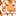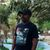# QlikView App Dev

Discussion Board for collaboration related to QlikView App Development.

cancel
Showing results for
Did you mean:Contributor

## Help me please__Why is the answer in the regular table not equal to the Pivot table?

Why is the answer in the regular table not equal to the Pivot table?

This my code in Expression Pivot table

=aggr(sum(DISTINCT(Fabric_QTY)*(ITEM_CONTAIN)/100),YARN_ITEM,Yarn_week)

Excample Yarn item = CVC20/1R week 186 RepliesMaster

I wasn't able to understand what you're trying to do here.

You are comparing two different formulas. Why should they give the same result?

Additionally, you are using Sum(Distinct ...) which is almost always a bad idea since the same value might repeat multiple times.MVP & Luminary

There are various things noticeable. At first the distinct within the sum() like orsh_ hinted. Further the dimensions within the second table aren't the same like in the aggr and then it missed an outer aggregation.

- MarcusContributor
Author

I tried the same formula with the table below. But the value does not match. So try to group with aggr. What formula should I use to calculate?MVP

Shouldn't you just use:

=sum(Fabric_QTY *(ITEM_CONTAIN/100))

for both tables?

-RobMaster

May be this:

=Aggr(Sum(Fabric_QTY * (ITEM_CONTAIN/100)),YARN_ITEM)MVP

Bala, why Aggr()?

-RobCommunity Browser